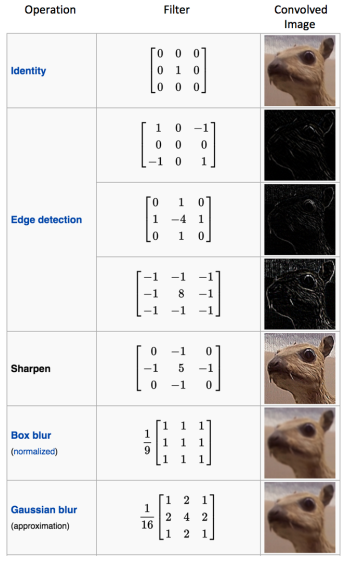2 views

I have been doing deep learning with CNN for a while and I realize that the inputs for a model are always squared images.

I see that neither convolution operation or neural network architecture itself require such property.

So, what is the reason for that?

by (108k points)

Convolution is the first layer in a Neural Network to extract features from an input image. Convolution helps in preserving the relationship between pixels by learning image features using small squares of input data. It is represented by a mathematical operation that takes two inputs such as image matrix and a filter or kernel.

Convolution of a picture with different filters will perform operations like edge detection, blur and sharpen by applying different-different filters.

The example below shows us various convolution image after applying different types of filters or Kernels.